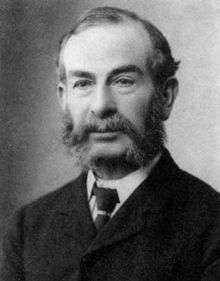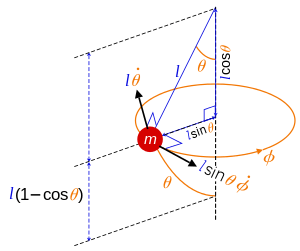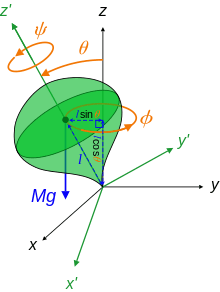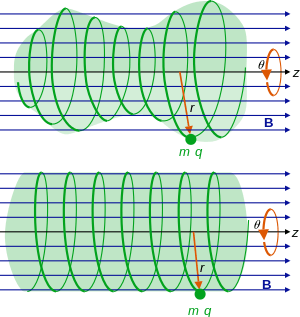# Routhian mechanicsEdward John Routh, 1831–1907.

In analytical mechanics, a branch of theoretical physics, Routhian mechanics is a hybrid formulation of Lagrangian mechanics and Hamiltonian mechanics developed by Edward John Routh. Correspondingly, the Routhian is the function which replaces both the Lagrangian and Hamiltonian functions.

The Routhian, like the Hamiltonian, can be obtained from a Legendre transform of the Lagrangian, and has a similar mathematical form to the Hamiltonian, but is not exactly the same. The difference between the Lagrangian, Hamiltonian, and Routhian functions are their variables. For a given set of generalized coordinates representing the degrees of freedom in the system, the Lagrangian is a function of the coordinates and velocities, while the Hamiltonian is a function of the coordinates and momenta.

The Routhian differs from these functions in that some coordinates are chosen to have corresponding generalized velocities, the rest to have corresponding generalized momenta. This choice is arbitrary, and can be done to simplify the problem. It also has the consequence that the Routhian equations are exactly the Hamiltonian equations for some coordinates and corresponding momenta, and the Lagrangian equations for the rest of the coordinates and their velocities. In each case the Lagrangian and Hamiltonian functions are replaced by a single function, the Routhian. The full set thus has the advantages of both sets of equations, with the convenience of splitting one set of coordinates to the Hamilton equations, and the rest to the Lagrangian equations.

Often the Routhian approach may offer no advantage, but one notable case where this is useful is when a system has cyclic coordinates (also called "ignorable coordinates"), by definition those coordinates which do not appear in the original Lagrangian. The Lagrangian equations are powerful results, used frequently in theory and practice, since the equations of motion in the coordinates are easy to set up. However, if cyclic coordinates occur there will still be equations to solve for all the coordinates, including the cyclic coordinates despite their absence in the Lagrangian. The Hamiltonian equations are useful theoretical results, but less useful in practice because coordinates and momenta are related together in the solutions - after solving the equations the coordinates and momenta must be eliminated from each other. Nevertheless, the Hamiltonian equations are perfectly suited to cyclic coordinates because the equations in the cyclic coordinates trivially vanish, leaving only the equations in the non cyclic coordinates.

The Routhian approach has the best of both approaches, because cyclic coordinates can be split off to the Hamiltonian equations and eliminated, leaving behind the non cyclic coordinates to be solved from the Lagrangian equations. Overall fewer equations need to be solved compared to the Lagrangian approach.

As with the rest of analytical mechanics, Routhian mechanics is completely equivalent to Newtonian mechanics, all other formulations of classical mechanics, and introduces no new physics. It offers an alternative way to solve mechanical problems.

## Definitions

In the case of Lagrangian mechanics, the generalized coordinates q1, q2, ... and the corresponding velocities dq1/dt, dq2/dt, ..., and possibly time[nb 1] t, enter the Lagrangian,where the overdots denote time derivatives.

In Hamiltonian mechanics, the generalized coordinates q1, q2, ... and the corresponding generalized momenta p1, p2, ..., and possibly time, enter the Hamiltonian,where the second equation is the definition of the generalized momentum pi corresponding to the coordinate qi (partial derivatives are denoted using ). The velocities dqi/dt are expressed as functions of their corresponding momenta by inverting their defining relation. In this context, pi is said to be the momentum "canonically conjugate" to qi.

The Routhian is intermediate between L and H; some coordinates q1, q2, ..., qn are chosen to have corresponding generalized momenta p1, p2, ..., pn, the rest of the coordinates ζ1, ζ2, ..., ζs to have generalized velocities 1/dt, 2/dt, ..., s/dt, and time may appear explicitly;

 Routhian (n + s degrees of freedom)where again the generalized velocity dqi/dt is to be expressed as a function of generalized momentum pi via its defining relation. The choice of which n coordinates are to have corresponding momenta, out of the n + s coordinates, is arbitrary.

The above is used by Landau and Lifshitz, and Goldstein. Some authors may define the Routhian to be the negative of the above definition.

Given the length of the general definition, a more compact notation is to use boldface for tuples (or vectors) of the variables, thus q = (q1, q2, ..., qn), ζ = (ζ1, ζ2, ..., ζs), p = (p1, p2, ..., pn), and d ζ/dt = (1/dt, 2/dt, ..., s/dt), so thatwhere · is the dot product defined on the tuples, for the specific example appearing here:## Equations of motion

For reference, the Lagrangian equations for s degrees of freedom are a set of s coupled second order ordinary differential equations in the coordinateswhere j = 1, 2, ..., s, and the Hamiltonian equations for n degrees of freedom are a set of 2n coupled first order ordinary differential equations in the coordinates and momentaBelow, the Routhian equations of motion are obtained in two ways, in the process other useful derivatives are found that can be used elsewhere.

### Two degrees of freedom

Consider the case of a system with two degrees of freedom, q and ζ, with generalized velocities dq/dt and /dt, and the Lagrangian is time-dependent. (The generalization to any number of degrees of freedom follows exactly the same procedure as with two). The Lagrangian of the system will have the formThe differential of L isNow change variables, from the set (q, ζ, dq/dt, /dt) to (q, ζ, p, /dt), simply switching the velocity dq/dt to the momentum p. This change of variables in the differentials is the Legendre transformation. The differential of the new function to replace L will be a sum of differentials in dq, , dp, d(/dt), and dt. Using the definition of generalized momentum and Lagrange's equation for the coordinate q:we haveand to replace pd(dq/dt) by (dq/dt)dp, recall the product rule for differentials,[nb 2] and substituteto obtain the differential of a new function in terms of the new set of variables:Introducing the Routhianwhere again the velocity dq/dt is a function of the momentum p, we havebut from the above definition, the differential of the Routhian isComparing the coefficients of the differentials dq, , dp, d(/dt), and dt, the results are Hamilton's equations for the coordinate q,and Lagrange's equation for the coordinate ζand taking the total time derivative of the second equation and equating to the first. Notice the Routhian replaces the Hamiltonian and Lagrangian functions in all the equations of motion.

The remaining equation states the partial time derivatives of L and R are negatives### Any number of degrees of freedom

For n + s coordinates as defined above, with Routhianthe equations of motion can be derived by a Legendre transformation of this Routhian as in the previous section, but another way is to simply take the partial derivatives of R with respect to the coordinates qi and ζj, momenta pi, and velocities j/dt, where i = 1, 2, ..., n, and j = 1, 2, ..., s. The derivatives areThe first two are identically the Hamiltonian equations. Equating the total time derivative of the fourth set of equations with the third (for each value of j) gives the Lagrangian equations. The fifth is just the same relation between time partial derivatives as before. To summarize

 Routhian equations of motion (n + s degrees of freedom)The total number of equations is 2n + s, there are 2n Hamiltonian equations plus s Lagrange equations.

## Energy

Since the Lagrangian has the same units as energy, the units of the Routhian are also energy. In SI units this is the Joule.

Taking the total time derivative of the Lagrangian leads to the general resultIf the Lagrangian is independent of time, the partial time derivative of the Lagrangian is zero, L/∂t = 0, so the quantity under the total time derivative in brackets must be a constant, it is the total energy of the system(If there are external fields interacting with the constituents of the system, they can vary throughout space but not time). This expression requires the partial derivatives of L with respect to all the velocities dqi/dt and j/dt. Under the same condition of R being time independent, the energy in terms of the Routhian is a little simpler, substituting the definition of R and the partial derivatives of R with respect to the velocities j/dt,Notice only the partial derivatives of R with respect to the velocities j/dt are needed. In the case that s = 0 and the Routhian is explicitly time-independent, then E = R, that is, the Routhian equals the energy of the system. The same expression for R in when s = 0 is also the Hamiltonian, so in all E = R = H.

If the Routhian has explicit time dependence, the total energy of the system is not constant. The general result iswhich can be derived from the total time derivative of R in the same way as for L.

## Cyclic coordinates

The Routhian formulation is useful for systems with cyclic coordinates, because by definition those coordinates do not enter L, and hence R. The corresponding partial derivatives of L and R with respect to those coordinates are zero, which equates to the corresponding generalized momenta reducing to constants. To make this concrete, if the qi are all cyclic coordinates, and the ζj are all non cyclic, thenwhere the αi are constants. With these constants substituted into the Routhian, R is a function of only the non cyclic coordinates and velocities (and in general time also)The 2n Hamiltonian equations in the cyclic coordinates automatically vanish,and the s Lagrangian equations are in the non cyclic coordinatesThus the problem has been reduced to solving the Lagrangian equations in the non cyclic coordinates, with the advantage of the Hamiltonian equations cleanly removing the cyclic coordinates.

If we are interested in how the cyclic coordinates change with time, the equations for the generalized velocities corresponding to the cyclic coordinates can be integrated.

## Examples

The Routhian method does not guarantee the equations of motion will be simple, however it will lead to fewer equations.

### Central potential in spherical coordinates

One general class of mechanical systems with cyclic coordinates are those with central potentials, because potentials of this form only have dependence on radial separations and no dependence on angles.

Consider a particle of mass m under the influence of a central potential V(r) in spherical polar coordinates (r, θ, φ)Notice φ is cyclic, because it does not appear in the Lagrangian. The momentum conjugate to φ is the constantin which r and /dt can vary with time, but the angular momentum pφ is constant. The Routhian can be taken to beWe can solve for r and θ using Lagrange's equations, and do not need to solve for φ since it is eliminated by Hamiltonian's equations. The r equation isand the θ equation isThe Routhian approach has obtained two coupled nonlinear equations. By contrast the Lagrangian approach leads to three nonlinear coupled equations, mixing in the first and second time derivatives of φ in all of them, despite its absence from the Lagrangian.

The r equation isthe θ equation isthe φ equation is### Symmetric mechanical systems

#### Spherical pendulumSpherical pendulum: angles and velocities.

Consider the spherical pendulum, a mass m (known as a "pendulum bob") attached to a rigid rod of length l of negligible mass, subject to a local gravitational field g. The system rotates with angular velocity /dt which is not constant. The angle between the rod and vertical is θ and is not constant.

The Lagrangian is[nb 3]and φ is the cyclic coordinate for the system with constant momentumwhich again is physically the angular momentum of the system about the vertical. The angle θ and angular velocity /dt vary with time, but the angular momentum is constant. The Routhian isThe θ equation is found from the Lagrangian equationsor simplifying by introducing the constantsgivesThis equation resembles the simple nonlinear pendulum equation, because it can swing through the vertical axis, with an additional term to account for the rotation about the vertical axis (the constant a is related to the angular momentum pφ).

Applying the Lagrangian approach there are two nonlinear coupled equations to solve.

The θ equation isand the φ equation is#### Heavy symmetrical topHeavy symmetric top in terms of the Euler angles.

The heavy symmetrical top of mass M has Lagrangianwhere ψ, φ, θ are the Euler angles, θ is the angle between the vertical z-axis and the top's z-axis, ψ is the rotation of the top about its own z-axis, and φ the azimuthal of the top's z-axis around the vertical z-axis. The principal moments of inertia are I1 about the top's own x axis, I2 about the top's own y axes, and I3 about the top's own z-axis. Since the top is symmetric about its z-axis, I1 = I2. Here the simple relation for local gravitational potential energy V = Mglcosθ is used where g is the acceleration due to gravity, and the centre of mass of the top is a distance l from its tip along its z-axis.

The angles ψ, φ are cyclic. The constant momenta are the angular momenta of the top about its axis and its precession about the vertical, respectively:From these, eliminating /dt:we haveand to eliminate /dt, substitute this result into pψ and solve for /dt to findThe Routhian can be taken to beand sincewe haveThe first term is constant, and can be ignored since only the derivatives of R will enter the equations of motion. The simplified Routhian, without loss of information, is thusThe equation of motion for θ is, by direct calculation,or by introducing the constantsa simpler form of the equation is obtainedAlthough the equation is highly nonlinear, there is only one equation to solve for, it was obtained directly, and the cyclic coordinates are not involved.

By contrast, the Lagrangian approach leads to three nonlinear coupled equations to solve, despite the absence of the coordinates ψ and φ in the Lagrangian.

The θ equation isthe ψ equation isand the φ equation is### Velocity-dependent potentials

#### Classical charged particle in a uniform magnetic fieldClassical charged particle in uniform B field, using cylindrical coordinates. Top: If the radial coordinate r and angular velocity /dt vary, the trajectory is a helicoid with varying radius but uniform motion in the z direction. Bottom: Constant r and /dt means a helicoid with constant radius.

Consider a classical charged particle of mass m and electric charge q in a static (time-independent) uniform (constant throughout space) magnetic field B. The Lagrangian for a charged particle in a general electromagnetic field given by the magnetic potential A and electric potential φ isIt is convenient to use cylindrical coordinates (r, θ, z), so thatIn this case the electric potential is zero, φ = 0, and we can choose the axial gauge for the magnetic potentialand the Lagrangian isNotice this potential has an effectively cylindrical symmetry (although it also has angular velocity dependence), since the only spatial dependence is on the radial length from an imaginary cylinder axis.

There are two cyclic coordinates, θ and z. The canonical momenta conjugate to θ and z are the constantsso the velocities areThe angular momentum about the z axis is not pθ, but the quantity mr2/dt, which is not conserved due to the contribution from the magnetic field. The canonical momentum pθ is the conserved quantity. It is still the case that pz is the linear or translational momentum along the z axis, which is also conserved.

The radial component r and angular velocity /dt can vary with time, but pθ is constant, and since pz is constant it follows dz/dt is constant. The Routhian can take the formwhere in the last line, the pz2/2m term is a constant and can be ignored without loss of continuity. The Hamiltonian equations for θ and z automatically vanish and do not need to be solved for. The Lagrangian equation in ris by direct calculationwhich after collecting terms isand simplifying further by introducing the constantsthe differential equation isTo see how z changes with time, integrate the momenta expression for pz abovewhere cz is an arbitrary constant, the initial value of z to be specified in the initial conditions.

The motion of the particle in this system is helicoidal, with the axial motion uniform (constant) but the radial and angular components varying in a spiral according to the equation of motion derived above. The initial conditions on r, dr/dt, θ, /dt, will determine if the trajectory of the particle has a constant r or varying r. If initially r is nonzero but dr/dt = 0, while θ and /dt are arbitrary, then the initial velocity of the particle has no radial component, r is constant, so the motion will be in a perfect helix. If r is constant, the angular velocity is also constant according to the conserved pθ.

With the Lagrangian approach, the equation for r would include /dt which has to be eliminated, and there would be equations for θ and z to solve for.

The r equation isthe θ equation isand the z equation isThe z equation is trivial to integrate, but the r and θ equations are not, in any case the time derivatives are mixed in all the equations and must be eliminated.

## Footnotes

1. The coordinates are functions of time, so the Lagrangian always has implicit time-dependence via the coordinates. If the Lagrangian changes with time irrespective of the coordinates, usually due to some time-dependent potential, then the Lagrangian is said to have "explicit" time-dependence. Similarly for the Hamiltonian and Routhian functions.
2. For two functions u and v, the differential of the product is d(uv) = udv + vdu.
3. The potential energy is actuallybut since the first term is constant, it can be ignored in the Lagrangian (and Routhian) which only depend on derivatives of coordinates and velocities. Subtracting this from the kinetic energy means a plus sign in the Lagrangian, not minus.

## References

• Landau, L. D.; Lifshitz, E. M. Mechanics (3rd ed.). Butterworth Heinemann. p. 134. ISBN 9780750628969.
• Hand, L. N.; Finch, J. D. Analytical Mechanics (2nd ed.). Cambridge University Press. p. 23. ISBN 9780521575720.
• Kibble, T. W. B.; Berkshire, F. H. (2004). Classical Mechanics (5th ed.). Imperial College Press. p. 236. ISBN 9781860944352.
• Goldstein, Herbert (1980). Classical Mechanics (2nd ed.). San Francisco, CA: Addison Wesley. pp. 352–353. ISBN 0201029189.
• Goldstein, Herbert; Poole, Charles P., Jr.; Safko, John L. (2002). Classical Mechanics (3rd ed.). San Francisco, CA: Addison Wesley. pp. 347–349. ISBN 0-201-65702-3.